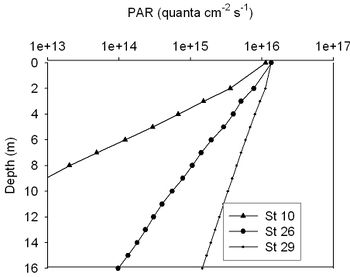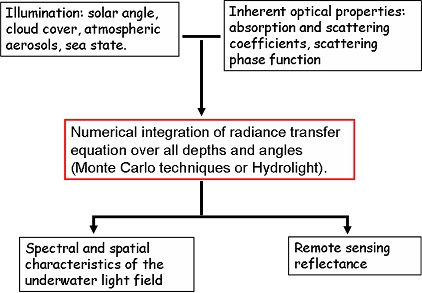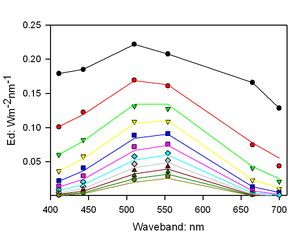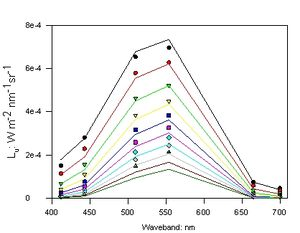# Light fields and optics in coastal waters

The quantitative study of underwater light fields in coastal waters and shelf seas has important applications in ecology, engineering and remote sensing. This article introduces the theoretical basis of optical measurements. Attention is paid to descriptors of light fields, optical properties of natural waters and light field modelling. For more information about the measurement of light fields, see also the article Optical measurements in coastal waters.

## Introduction of underwater light fields

### Relevance

The quantitative study of underwater light fields in coastal waters and shelf seas has important applications in ecology, engineering and remote sensing. Underwater light fields:

1. play an important role in determining rates of photosynthesis by phytoplankton and macrophytes, and therefore set limits on the productivity of marine ecosystems.
2. influence the range at which objects are visible under water, which is of great significance for diving and engineering operations and also for visual interactions between predators and prey species.
3. determine the volume reflectance of seawater which is important for optical remote sensing.

### Dynamics of underwater light fields

Variations in underwater light fields originate from changes in both solar illumination and seawater composition, and predicting this variability involves the study of radiative transfer in an optically complex medium. There is an extensive literature on underwater light fields and associated problems in marine optics, but the fundamental concepts are clearly laid out in the books by Kirk (1994), Mobley (1994) and Bukata et al (1995). One of the challenges posed by coastal light fields is the potential for interaction and between physical and biological factors. For example, sediment particles in suspension can limit the illumination of benthic photosynthetic organisms, but the presence of those organisms may stabilise the sediment surface and reduce the probability of sediment re-suspension taking place.

Underwater light fields are vulnerable to human activities. Anthropogenic disturbances such as eutrophication, dredging operations and accelerated soil erosion due to the de-afforestation of river catchments can modify underwater light climates in coastal regions. Increases in turbidity produced by these activities can damage important ecosystem components such as coral reefs and sea grass beds.

The underwater light field depends on:

1. the optical characteristics of sea water: The optical characteristics of coastal waters are determined not only by water itself, but also by suspended particulate material (minerals, organic detritus and phytoplankton) and coloured dissolved organic matter. The presence of relatively high concentrations of dissolved organic matter or minerals often distinguishes coastal waters from those of deeper seas, and accounts for their green or brown colouration.
2. the conditions of illumination: The conditions of illumination depend on solar angle, the degree and type of cloud cover, and the state of the sea surface. As a result, underwater light fields show strong diurnal and seasonal variability.

## Light field descriptors

Radiance is the fundamental quantity in light field measurement. It is usually denoted $L(q,f,\lambda)$ where the angles $q$ (zenith) and $f$ (azimuth) specify the direction in which the radiance is measured and $\lambda$ is the wavelength. The units of radiance are W m-2 nm-1 sr-1. Measurements of the water-leaving radiance $L_w$ just above the surface at $q = 0$ (nadir viewing) are particularly important for remote sensing.

Planar and scalar irradiances $E_x(\lambda)$ are generated by integrating radiances over defined intervals of solid angle $D_w$. They have units of W m-2 nm-1. For planar irradiances, the radiances are multiplied by the cosine of their angle of incidence on the detecting plane before carrying out the integration. Scalar irradiances omit the cosine weighting. Measurements of planar irradiances are made using a diffusing disc as a collector, while scalar irradiances are measured use a diffusing sphere. Commonly used irradiances are summarized Table 1.

Quantity Name Angular limits
$E_{\theta}(\lambda)$ Scalar irradiance whole sphere
$[E_{\theta}(\lambda]_u$ Upward scalar irradiance lower hemisphere
$[E_{\theta}(\lambda]_d$ Downward scalar irradiance upper hemisphere
$E_u(\lambda)$ Upward planar irradiance lower hemisphere
$E_d(\lambda)$ Downward planar irradiance upper hemisphere

A full description of an underwater light field would involve the specification of the spectral and angular distribution of radiance as a function of depth and geographical location, but this degree of detail is impractically cumbersome. The quantities most frequently specified are upward radiances and upward and downward irradiances.

### Wavelength ranges

Studies of underwater light fields are usually contained within a waveband of approximately 300-900 nm: outside these boundaries, absorption by water strongly limits light penetration. Photosynthesis and animal vision mainly utilise the wavelength region of 400-700 nm, but damaging radiation can affect photosynthetic organisms at ultra-violet wavelengths (300-400 nm) while the near infra red region (700-900 nm) is important for remote sensing applications.

### Measurement units

Three systems of units may be encountered in the literature on underwater light fields:

1. Radiometric units: In physical radiometry, light energy is measured in joules (J), radiometric power in watts (W or J s-1), and the source brightness as power per unit solid angle (W sr-1).
2. Quantum units: In photochemistry and photobiology, where molecular events are driven by the absorption of individual quanta, it is often useful to measure light fields in terms of numbers of photons rather than energy. A convenient unit is the mol (Avogadro’s Number) of photons. In the photobiology literature, one mol of photons is often referred to as an einstein, but the unit is redundant. The energy associated with a single photon of a given wavelength in vacuo is $e = hc/\lambda$, where $h$ is Planck’s constant and $c$ the speed of light. The inverse wavelength dependence means that conversion between radiometric and quantum units (for example from W m-2 s-1 to mol photons m-2 s-1 in the case of irradiance) requires explicit knowledge of the spectral distribution of the light field. Integration of scalar quantum irradiance across the visible spectrum (400-700 nm) gives a measure of photosynthetically active radiation (PAR: mol photons m-2 s-1).
3. Photometric units: The visual effect of a given source of illumination depends on the spectral sensitivity of the human eye. This leads to a system of photometric units based on the lumen as the measurement of effective power, and is discussed in DeCusatis (1997). Conversion between radiometric and photometric units has to take both the standard eye response curve and the spectral distribution of the light field into account.

## Optical properties of natural waters

This section describes the inherent optical properties (IOPs) op natural waters. The total IOP of water is its own contribution and four types of optically significant constituents.

### Inherent optical properties (IOPs)

The optical characteristics of a light-transmitting medium can be specified in terms of its inherent optical properties, which represent the effect of an optically thin slab of the medium on the transmission of a collimated light beam. The main IOPs are summarized in table 2:

 $a$ absorption coefficient m-1 $b$ scattering coefficient m-1 $c = a + b$ attenuation coefficient m-1 $\beta$ scattering phase function sr-1

Formal definitions for these IOPs may be found in Mobley (1994). The absorption, scattering and attenuation coefficients have fairly obvious meanings, while the phase function is a measure of the relative angular distribution of the scattered light. The scattering coefficient (b) is often partitioned into forward and backwards components, and the backscattering coefficient (bb) is of great importance for determining the remote sensing reflection of a water body.

The total IOPs of a body of seawater can be considered as the sum of the partial contributions from water itself and a number of optically significant constituents. These constituents are generally divided into four classes:

1. Phytoplankton cells and colonies (Phyt)
2. Mineral suspended solids (MSS)
3. Coloured dissolved organic matter (CDOM)
4. Organic suspended solids or detritus (OSS)

The concentration of the photosynthetic pigment chlorophyll a (Chl, mg m-3) is often used as a proxy variable for phytoplankton biomass. Mineral particle and organic detritus concentrations (g m-3) are determined by filtering water samples through a fine glass fibre filter and measuring the dry weight of material retained before and after combusting the filter at 500°C to remove the organic portion. Since there is no standard method for measuring the mass concentration of coloured dissolved organic matter, it is conventionally measured as the absorption coefficient at 440 nm of seawater which has been passed through a membrane filter with a 0.2 μm pore size.

The total absorption coefficient can be written as the sum of the contributions of the individual constituents:

$a_{total} = a_{water}\, +\, a_{Phyt}\, +\, a_{MSS}\, +\, a_{CDOM}\, +\, a_{OSS}$

The scattering coefficient ($b$), attenuation coefficient ($c$) and phase function ($\beta$) can all be partitioned in a similar manner. In practice, however, the optical contributions of these four classes of material may not be completely distinct: blooms formed by phytoplankton groups such as coccolithophores and diatoms may generate significant concentrations of mineral particles (calcite and silica respectively), and organic detrital material may form flocs which incorporate suspended minerals.

### Specific optical properties and characteristics of constituents

The optical contributions of the main classes of constituents can be calculated by multiplying the concentrations of each constituent by the value of its inherent properties expressed per unit concentration. The latter quantity is often referred to as a specific inherent optical property or specific optical cross section. For example:

$a_{MSS} = MSS \times a^*(MSS)$

where $MSS$ is the concentration of suspended minerals and $a^*(MSS)$ is the specific absorption cross section for this constituent, with units of m2 g-1. The convention that seawater contains four classes of optically significant constituents tends to conceal the fact that there can be considerable variability in specific optical properties within each class of constituent. Moreover, optical characterisation of the four main classes of constituent is currently incomplete.

Phytoplankton cells and colonies (Phyt): A great deal of effort has been devoted to the study of the absorption and scattering characteristics of phytoplankton cells (see for example Sathyendranath et al 1987, Bricaud et al 1988 and Johnsen et al 1994). The absorption spectra generally show maxima around the main chlorophyll absorption peaks at 440 nm and 675 nm, with subsidiary features depending on the presence of accessory light harvesting and photoprotective pigments. Phytoplankton scattering poses considerable practical and theoretical challenges because of the wide range of size and structure exhibited by individual cells and colonies. A helpful review of the theoretical problems is given by Quirantes and Bernard (2004) and measurement issues are discussed in Volten et al.(1998). For all but the smallest species, phytoplankton scattering is strongly peaked in the forward direction. One interesting feature in the total scattering spectra is the presence of minima near the chlorophyll absorption peaks which is due to Kettler-Helmholtz anomalous dispersion. There is some evidence that the specific backscattering coefficients of most phytoplankton species are too low to account for the magnitude of water-leaving radiance signals observed from natural populations, but measurements in this area are imprecise and current theoretical models do not take the full structural complexity of phytoplankton cells into account.
Mineral suspended solids (MSS): The optics of some types of mineral particles have been systematically explored (Babin and Stramski 2005). Their absorption spectra are generally high in the blue wavebands and decrease towards the red, and for field samples their shape is often similar to that for CDOM. Scattering characteristics are influenced by size distribution and refractive index (Wozniak and Stramski 2004). It is not clear whether laboratory samples of mineral dusts are representative of all the materials found in coastal locations.
Coloured dissolved organic matter (CDOM) CDOM absorption has been extensively characterised. It is generally described by an exponential function of the form: $a(\lambda\,)_{cdom}=a(\lambda_{\theta})_{cdom}\, e^{-S(\lambda - \lambda_{\theta})}$. In which: $\lambda_{\theta}$ is a reference wavelength (usually 440 nm) and $S$ is a coefficient which has a typical value of 0.01 to 0.02 in UK coastal waters (Bricaud et al 1981). Light scattering by CDOM is generally assumed negligible, but it is possible that some of the coloured material passing through 0.2 μm filters is colloidal in nature and therefore capable of contributing to the total scattering coefficient.
Organic suspended solids or detritus (OSS): The optical properties of organic detritus are not well characterised. This is due to the wide range of material which falls under this classification, ranging from scenescent phytoplankton cells to faecal pellets in offshore waters and including terrestrial vegetable matter in estuaries. There is also the possibility that organic matter may form flocs which include mineral particles in estuaries and in post-bloom shelf-sea conditions.

### Other relevant parameters

Changes in light field parameters with depth ($z$) are often approximately exponential in nature. For example, the attenuation of downward planar irradiance in a uniform water column can be written:

$E_d(\lambda, z) = E_d (\lambda, \theta)e^{-K_d z}$,

in which $K_d$ is the vertical attenuation coefficient. Quantities such as $K_d$ are relatively insensitive to input conditions at moderate solar angles and are frequently referred to as apparent optical properties (AOPs) (Kirk 1994). Some examples of AOPs are:

• The attenuation coefficient for downwards planar irradiance ($K_d$)
• The attenuation coefficient for scalar irradiance ($K_{\theta}$)
• Irradiance reflectance ($R$)
• Remote sensing reflectance (measured just above the sea surface) ($R_{rs}$)
• The mean cosine ($\mu$)Figure 1: Measurements of PAR at three contrasting stations in the Irish Sea in April (station 10) and November (stations 26 and 29)

Photosynthetically available radiation (PAR) is the integral of scalar irradiance, expressed in quantum units, over the 400 -700 nm waveband. The term $K_{PAR}$, defined by analogy with $K_{\theta}$ and $K_d$, is sometimes used to describe the attenuation of PAR. However since the attenuation of light by seawater varies with wavelength, the reduction in PAR with depth can deviate from the exponential approximation in the upper part of the water column. Figure 1 shows the measured variability of PAR with depth for three contrasting stations in the Irish Sea.

The optical depth $z(\lambda;l)$ is defined as the depth at which $E_d$ is reduced to 1/e (~0.37) of its value immediately below the surface. This quantity is wavelength-dependent, as might be expected from the fact that the spectral distribution of the underwater light field changes with depth. The maximum depth at which significant photosynthesis can occur (the euphotic depth, $z_{eu}$) is conventionally taken to be the point at which PAR is reduced to 1% of its surface value (Kirk 1994).

The horizontal range of visibility ($y$) at which a black target is judged to be visible to a human observer is empirically related to the attenuation coefficient $c$ by the relationship:

$y\, = 4.8 / c$ .

A theoretical basis for this relationship, based on radiance transfer theory, is given by Zaneveld and Pegau (2003).

## Radiative transfer theory and light field modelling

The propagation of light energy through a medium which absorbs, scatters and contains internal sources is determined by the radiative transfer equation, which is widely used in fields such as astronomy and atmospheric science as well as hydrological optics (Goody and Yung 1989). In marine light fields, sunlight enters from above and is attenuated with depth ($z$). In the simple case where bottom reflectance can be neglected and the water is horizontally homogeneous, the change in monochromatic radiance in a given direction ($q,f$) as a function of depth ($z$) is given by

$\Large\frac{d L(z,q,f)}{dr}\normalsize = -cL(z,q,f) + L^*(z,q,f)+ S(z,q,f)$,

in which: $L^*(z,q,f)$ represents the gain in radiance due to light scattered from adjacent paths and $S(z,q,f)$ accounts for any internal sources. In coastal waters, the most important internal source terms arise from Raman scattering by water molecules and fluorescence from dissolved organic matter and phytoplankton pigments. For light-field modelling the radiative transfer equation is solved numerically using either Monte Carlo methods or more conceptually sophisticated mathematical techniques (Mobley 1994, Thomas and Stamnes 1999). The discrete ordinates method is commonly used in optical oceanography and is implemented in the commercially available Hydrolight software package (Sequoia Scientific). In order to construct a light field model, it is necessary to specify the nature of the input illumination, the IOPs of the medium, and any internal source functions (Figure 2). Light field models can generate a complete set of radiance values, but their output is usually summarised as a set of AOPs and reflectances which corresponds to those most frequently measured.Figure 2: Input/output relationships for the numerical modelling of radiative transfer in shelf seas.

### Optical closure

Ideally, it should be possible to construct a light field model of a coastal water column using measured values of the IOPs which would precisely replicate radiometric measurements made at the same time. This is known as ‘optical closure’. It is difficult to achieve in practice for two main reasons. First, it places great demands on the appropriate calibration and deployment of the measuring instruments. Second, some key IOPs such as the scattering phase function are difficult to measure in situ, and are usually estimated from the backscattering ratio (bb/b) using analytical functions. Figure 3 shows a typical attempt to achieve optical closure for a shelf sea station in the Irish Sea with constituent concentrations of: Chl 1.6 mg m-3, MSS 1.9 mg l-1, CDOM a440 0.2m-1. In this instance the match between measured and modelled $E_d$ values is reasonably good, but the $L_u$ plots diverge significantly below 5 m. The symbols in Figure 3 represent measurements made with a profiling radiometer (Satlantic SPMR) and are spaced at 1 m intervals from the sea surface. The lines indicate the output of Hydrolight calculations of downward irradiance (upper plot) and upward radiance (lower plot) which used in-situ measurements of IOPs as inputs. Absorption and scattering coefficients were measured using a dual tube photometer (Wet Labs ac-9) and backscattering coefficients measured using a Hydroscat 2 (HobiLabs) backscattering meter.